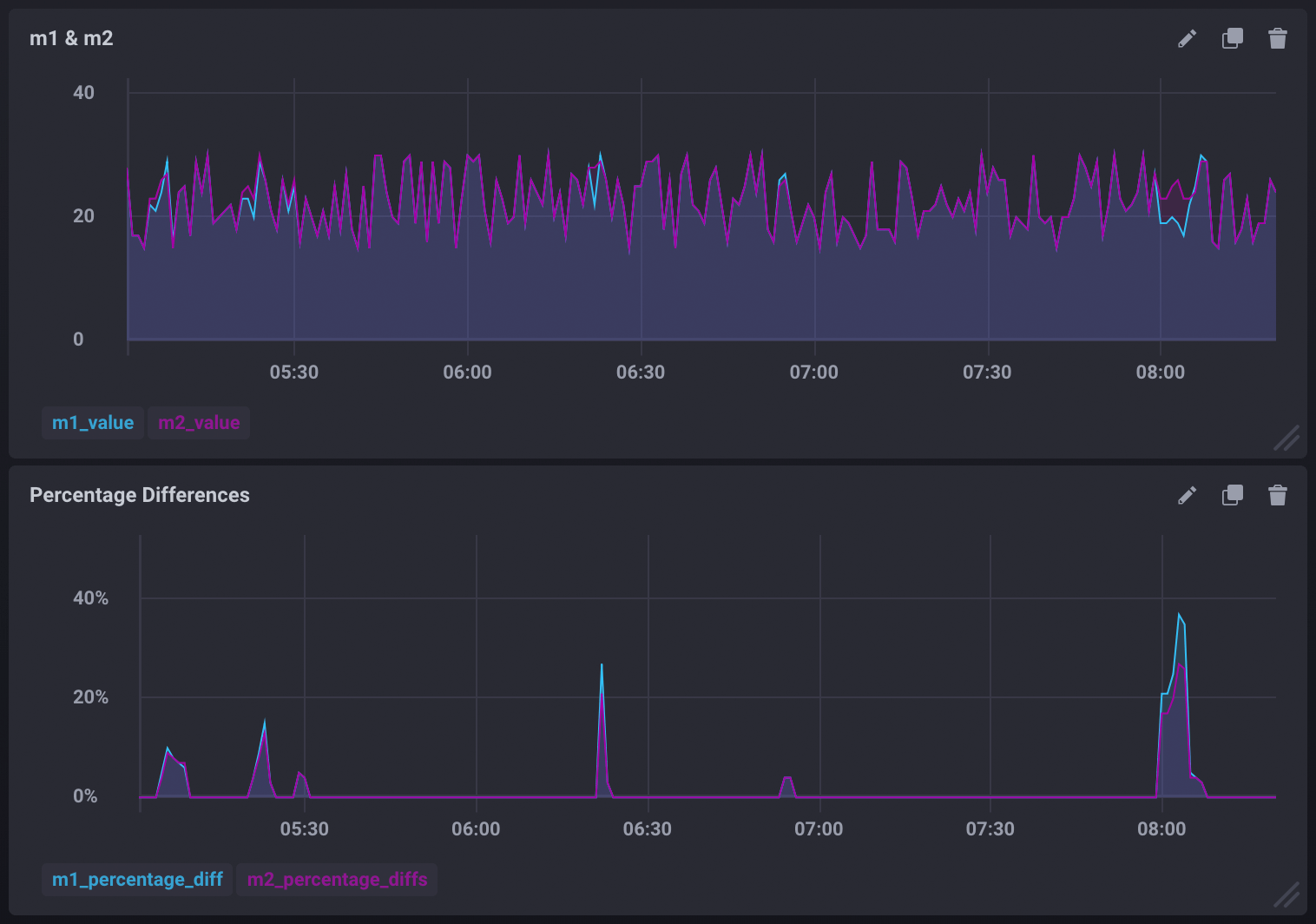# Trigger alerts by comparing two measurements

This page documents an earlier version of Kapacitor. Kapacitor v1.6 is the latest stable version. View this page in the v1.6 documentation.

Kapacitor allows you to create alerts based on two or more measurements. In this guide, we are going to compare two measurements, `m1` and `m2`, and create an alert whenever the two measurements are different. As an added bonus, we’ll also include a query that can be used to graph the percentage difference between the two measurements.

## Comparing measurements and creating an alert

The following TICKscript streams the `m1` and `m2` measurements, joins them, compares them, and triggers an alert if the two measurements are different.

``````var window_size = 1m

// Stream m1
var m1 = stream
|from()
.measurement('m1')
|window()
.period(window_size)
.every(window_size)
.align()
|count('value')
.as('value')

// Stream m2
var m2 = stream
|from()
.measurement('m2')
|window()
.period(window_size)
.every(window_size)
.align()
|count('value')
.as('value')

// Join m1 and m2
var data = m1
|join(m2)
.as('m1', 'm2')

// Compare the joined stream and alert when m1 and m2 values are different
data
.crit(lambda: "m1.value" != "m2.value")
.message('values were not equal m1 value is {{ index .Fields "m1.value" }} m2 value is {{ index .Fields "m2.value" }}')
``````

## Graphing the percentage difference between the measurements

Use the `data` stream defined in the TICKscript above to calculate the difference between `m1` and `m2`, transform it into a float, divide that difference by the actual values of `m1` and `m2`, then multiply them by 100. This will give you the percentage difference for each. Store the difference as new fields in the `diffs` measurement:

``````data
// Calculate the difference between m1 and m2
|eval(lambda: "m1.value" - "m2.value")
.as('value_diff')
.keep()
// Calculate the % difference of m1 and m2
|eval(lambda: (float("value_diff") / float("m1.value")) * 100.0, lambda: (float("value_diff") / float("m2.value")) * 100.0)
.as('diff_percentage_m1', 'diff_percentage_m2')
// Store the calculated differences in the 'diffs' measurement
|influxDBOut()
.measurement('diffs')
.database('mydb')
.create()
``````

This can be used to create visualizations similar to:## The full TICKscript

Below is the entire, uncommented TICKscript:

``````var window_size = 1m

var m1 = stream
|from()
.measurement('m1')
|window()
.period(window_size)
.every(window_size)
.align()
|count('value')
.as('value')

var m2 = stream
|from()
.measurement('m2')
|window()
.period(window_size)
.every(window_size)
.align()
|count('value')
.as('value')

var data = m1
|join(m2)
.as('m1', 'm2')

data
.crit(lambda: "m1.value" != "m2.value")
.message('values were not equal m1 value is {{ index .Fields "m1.value" }} m2 value is {{ index .Fields "m2.value" }}')

data
|eval(lambda: "m1.value" - "m2.value")
.as('value_diff')
.keep()
|eval(lambda: (float("value_diff") / float("m1.value")) * 100.0, lambda: (float("value_diff") / float("m2.value")) * 100.0)
.as('diff_percentage_m1', 'diff_percentage_m2')
|influxDBOut()
.measurement('diffs')
.database('mydb')
.create()
``````